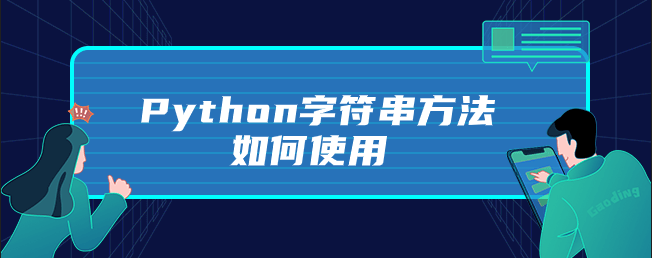# Python字符串方法如何使用1、find方法可以在一个较长的字符串中查找子串。

```a = 'abcdefghijk'
print(a.find('abc'))                                  #the result ： 0
print(a.find('abc',10,100))                           #the result ： 11  指定查找的起始和结束查找位置```

2、join方法是非常重要的字符串方法。

```a = ['1','2','3']
print('+'.join(a))                                    #the result ： 1+2+3```

3、split是join的逆方法。

`print('1+2+3+4'.split('+'))                          #the result ： ['1', '2', '3', '4']`

4、strip方法返回去除首位空格。

`print("   test   test    ".strip())                  #the result ：“test   test”`

replace方法返回某字符串。

`print("This is a test".replace('is','is_test'))     #the result ： This_test is_test a test`
Python开发开发项目